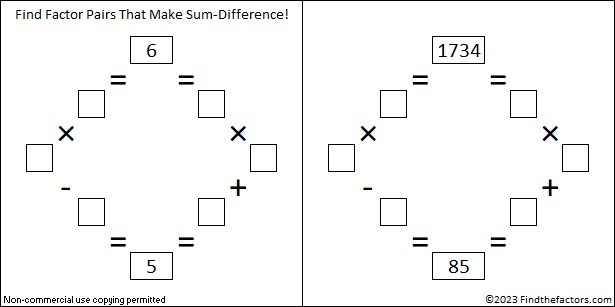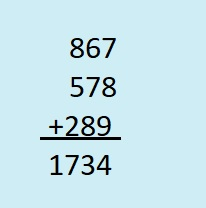# Both17 and 34 are Factors of 1734 and Help It Make Sum-Difference

Contents

### Today’s Puzzle:

1734 has only 6 factor pairs, but two of those pairs will solve a Sum-Difference puzzle.

Let’s start with the first puzzle below. Can you find a factor pair of 6 that adds up to 5 and another one that subtracts to 5? If you can, then you have solved the first puzzle. The second puzzle is really just a multiple of the first puzzle. 5 × what = 85? Multiply the numbers you write in the first puzzle by that same number, and you will have the numbers needed to solve the second puzzle. You can also look at the factor pairs in the next section to help you find the needed factors that sum or subtract to 85.### Factors of 1734:

Because 17×2=34, their concatenation, 1734, is divisible by three. All of the following concatenated numbers are also divisible by three: 12, 24, 36, 48, 510, 612, 714, 816, 918, 1020, 1122, 1224, 1326, 1428, 1530, 1632, 1734, 1836, 1938, and so forth.

• 1734 is a composite number.
• Prime factorization: 1734 = 2 × 3 × 17 × 17, which can be written 1734 = 2 × 3 × 17².
• 1734 has at least one exponent greater than 1 in its prime factorization so √1734 can be simplified. Taking the factor pair from the factor pair table below with the largest square number factor, we get √1734 = (√289)(√6) = 17√6.
• The exponents in the prime factorization are 1, 1, and 2. Adding one to each exponent and multiplying we get (1 + 1)(1 + 1)(2 + 1) = 2 × 2 × 3 = 12. Therefore 1734 has exactly 12 factors.
• The factors of 1734 are outlined with their factor pair partners in the graphic below.### More About the Number 1734:

Did you notice that both 17 and 34 are factors of 1734?

From OEIS.org we learn that 1734 has three factors that have 8 as one of their digits, 867, 578, and 289. What do you get when you add those factors together?1734 is the hypotenuse of two Pythagorean triples:
816-1530-1734, which is (8-15-17) times 102. and
966-1440-1734, which is 6 times (161-240-289).

1734 looks interesting in some other bases:
It’s 600 in base 17 because 6(17²) = 1734, and
it’s 369 in base 23 because 3(23²) + 6(23) + 9(1) = 1734.

What is the greatest common factor of 1734 and 2023? What is the least common multiple? The easiest way to know is to look at their prime factorizations.
1734 = 2·3·17²,
2023 = 7·17².
The greatest common factor is what they have in common: 17² = 289.
The least common multiple is 2·3·7·17² = 12138.

This site uses Akismet to reduce spam. Learn how your comment data is processed.